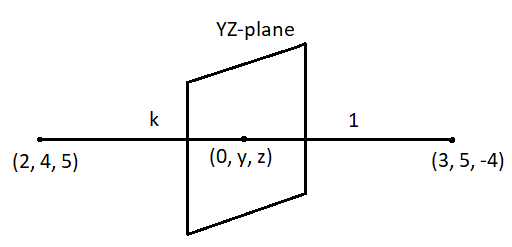The ratio in which the line joining points $\left( {2,4,5} \right)$ and $\left( {3,5, - 4} \right)$ divide ${\text{YZ}}$-plane isA.$- 2:3$B.$2:3$C.$- 3:2$D.$3:2$Verified
119.1k+ views
Hint: We know that on the ${\text{YZ}}$-plane the $x$-coordinate of the point that divides the plane will be zero. Assume the ratio that divides the given plane and use the section formula of 3D geometry to find the ratio by comparing the coordinates.

Formula used:
Let us consider two points $\left( {{x_1},{y_1},{z_1}} \right)$ and $\left( {{x_1},{y_1},{z_1}} \right)$ on a plane. The coordinates of a point $\left( {x,y,z} \right)$ that divides the line segment joining these two points in the ratio $m:n$ is given by:
$\left( {x,y,z} \right) = \left( {\frac{{m{x_2} + n{x_1}}}{{m + n}},\frac{{m{y_2} + n{y_1}}}{{m + n}},\frac{{m{z_2} + n{z_1}}}{{m + n}}} \right)$

Let us assume that the line segment joining the points $\left( {2,4,5} \right)$ and $\left( {3,5, - 4} \right)$ is divided in the ratio $k:1$ by the ${\text{YZ}}$-plane.
Substitute all the values in the section formula.
$\left( {x,y,z} \right) = \left( {\frac{{3k + 2}}{{k + 1}},\frac{{5k + 4}}{{k + 1}},\frac{{ - 4k + 5}}{{k + 1}}} \right)$
We know that on the ${\text{YZ}}$-plane the $x$-coordinate of the point will be zero. So, the coordinates of the points that divide the plane is $\left( {0,y,z} \right)$.Substitute $0$ for $x$.
$\left( {0,y,z} \right) = \left( {\frac{{3k + 2}}{{k + 1}},\frac{{5k + 4}}{{k + 1}},\frac{{ - 4k + 5}}{{k + 1}}} \right)$
Now, compare the coordinates to find the value of $k$.
$\Rightarrow 0 = \dfrac{{3k + 2}}{{k + 1}}$
$\Rightarrow 0 = 3k + 2$
$\Rightarrow 3k = - 2$
$\Rightarrow k = - \dfrac{2}{3}$
The required ratio can be calculated as:
$\Rightarrow - \dfrac{2}{3}:1 = - 2:3$
Therefore, the ratio in which the line joining points $\left( {2,4,5} \right)$ and $\left( {3,5, - 4} \right)$ divide ${\text{YZ}}$-plane is $- 2:3$.
So, the option (A) is the correct answer.

Note: As the line segment divides the ${\text{YZ}}$-plane this means that the line joining the given points is parallel to $x$-axis. So, its $x$-axis coordinate is $0$.
Make sure that the coordinates of the point that divides the plane is $\left( {0,y,z} \right)$. Do not consider it as $\left( {x,0,0} \right)$ otherwise this may lead to the incorrect answer.